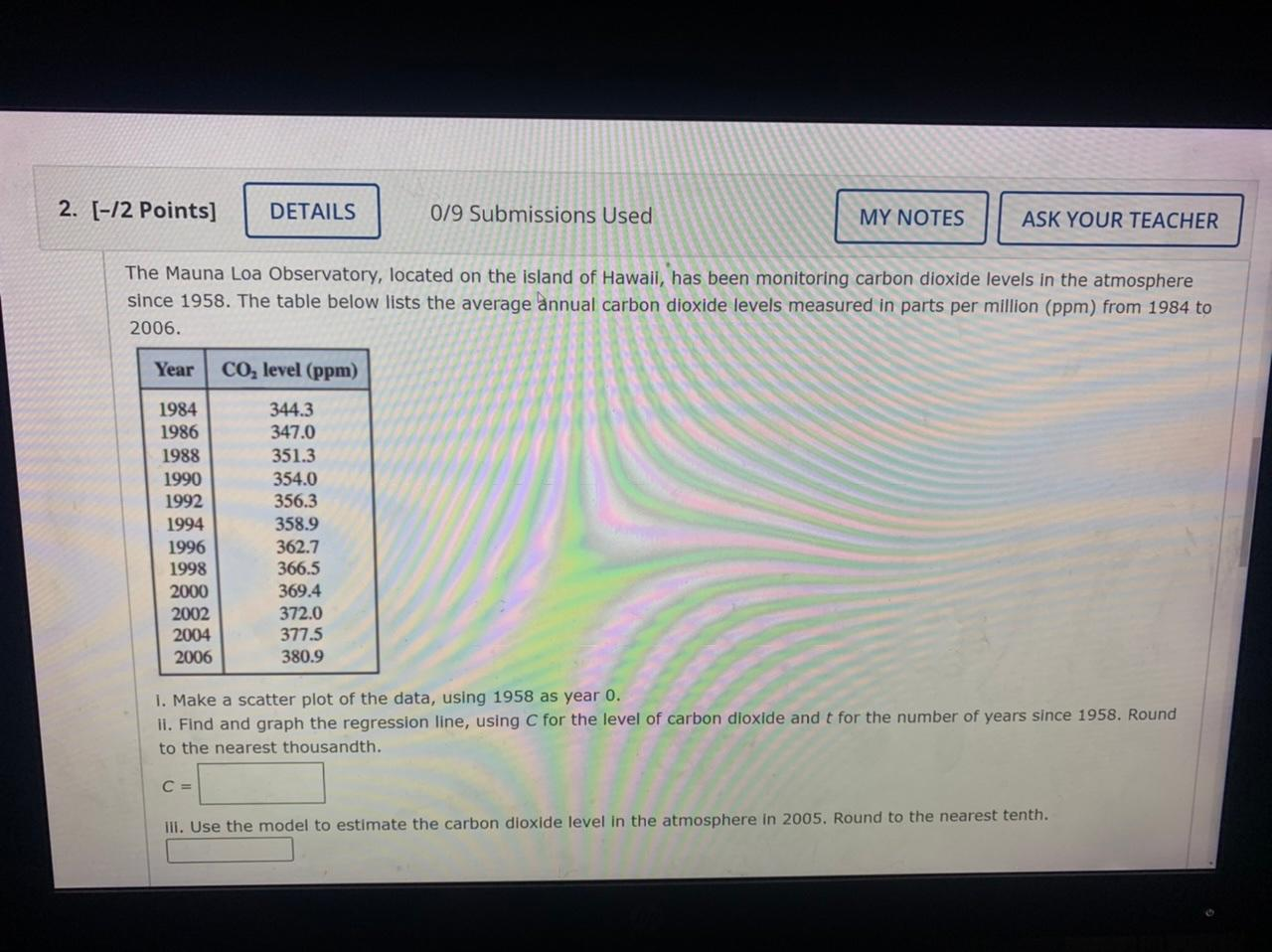# (Solved): Please answer this The Mauna Loa Observatory, located on the island of Hawaii, has been monitori ...The Mauna Loa Observatory, located on the island of Hawaii, has been monitoring carbon dioxide levels in the atmosphere since 1958. The table below lists the average annual carbon dioxide levels measured in parts per million (ppm) from 1984 to 2006. I. Make a scatter plot of the data, using 1958 as year 0. ii. Find and graph the regression line, using for the level of carbon dioxide and for the number of years since 1958 . Round to the nearest thousandth. III. Use the model to estimate the carbon dioxide level in the atmosphere in 2005. Round to the nearest tenth.

We have an Answer from Expert

SOLUTION:-

I. Make a scatter plot of the data, using 1958 as year 0.

(ii)

Let the required least square line is.

Normal equation are